## Filters

Sort by :
Clear All
Q
No potential energy is not negative when you displace particle against gravitation.  Take help of vectors for understanding this, Now if you are displacing particle against gravity that means you are taking particle in upward direction while the direction of g is in downward. So the dot product will give positive value equal to mgh.
Engineering
280 Views   |

The variation of acceleration due to gravity g with distance d from centre of the earth is best represented by

• Option 1)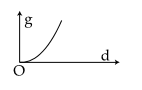• Option 2)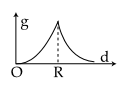• Option 3)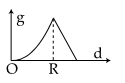• Option 4)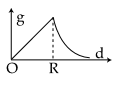As we learnt in Value of 'g' at ∞ -   if   Value of acceleration due to gravity height above earth's surface   - wherein No effect of earth gravitational pull at infinite distance.   if                                i.e.  Option 1) Incorrect Option 2) Incorrect Option 3) Incorrect Option 4) Correct
Engineering
377 Views   |

If the Earth has no rotational motion, the weight of a person on the equator is W. Determine the speed with which the earth would have to rotate about its axis so that the person at the equator will weighW.  Radius of the Earth is 6400 km and g=10 m/s2.

• Option 1)

• Option 2)

• Option 3)

• Option 4)

As we learnt in Variation in 'g' due to Rotation of earth - latitude angle Angular velocity of rotation of earth New value of 'g'.     - wherein Apparent weight of body decrease with rotation of earth so value of g also decrease.     At equator  Option 1) 1.1×10−3 rad/s Incorrect Option 2)  0.83×10−3 rad/s Incorrect Option 3)  0.63×10−3 rad/s Correct Option 4)  0.28×10−3 rad/s   Incorrect
Engineering
151 Views   |

The mass density of a spherical body is  given by ρ (r)=for r ≤ R and ρ (r)=0 for r > R, where r is the distance from the centre. The correct graph that describes qualitatively the acceleration, a, of a test particle as a function of r is

• Option 1)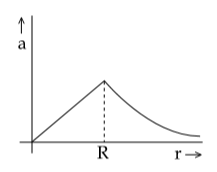• Option 2)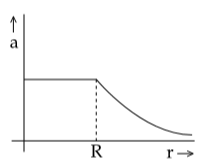• Option 3)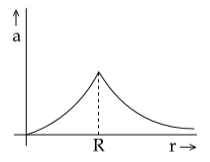• Option 4)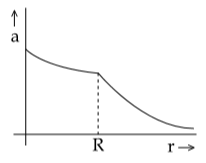As we learnt in Gravitational field Intensity -     - wherein    Given that  of spherical body  inside the surface of sphere intensity                                                 or  Option 1) Incorrect Option 2) Correct Option 3) Incorrect Option 4) Incorrect
Exams
Articles
Questions